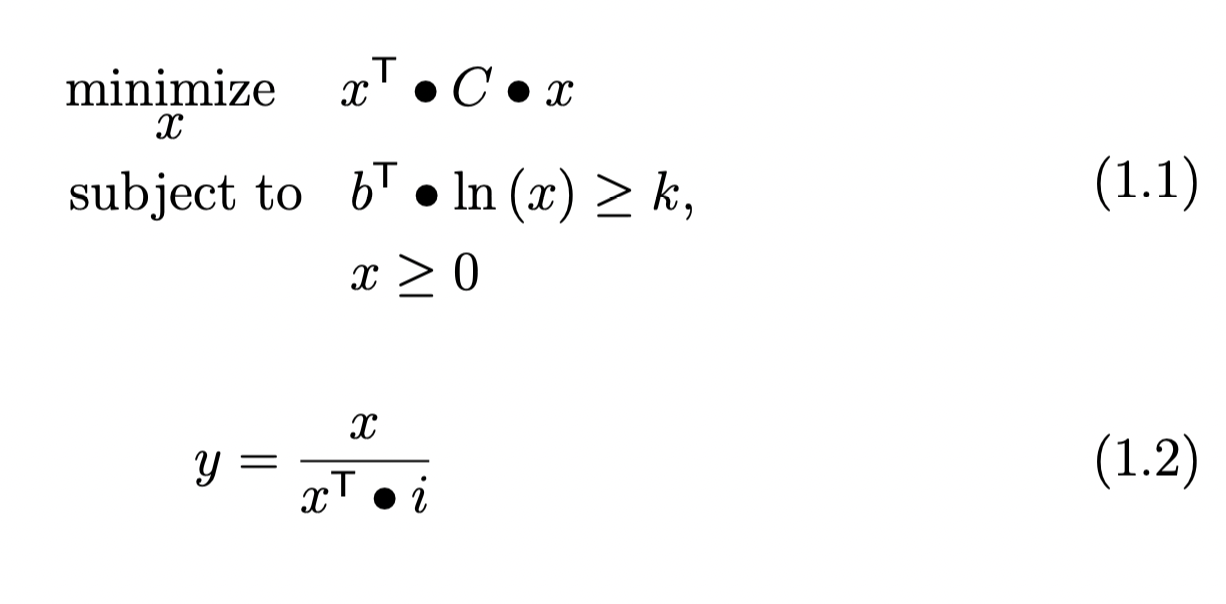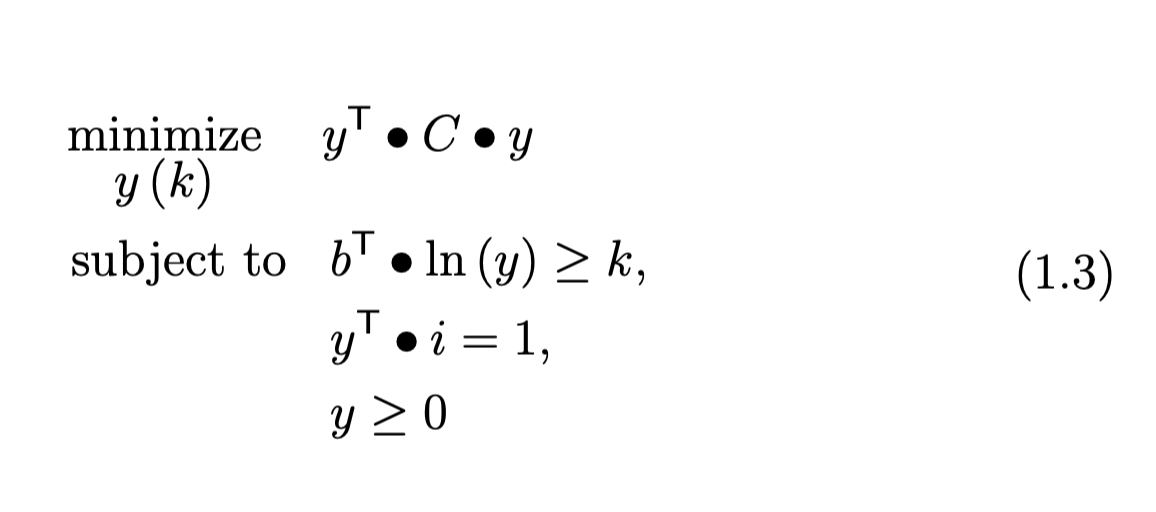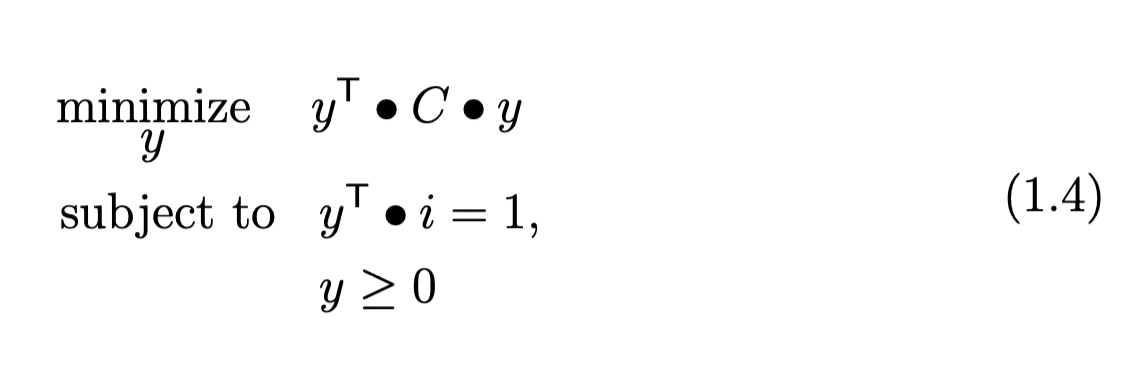# #StackBounty: #python #numpy #optimization #cvxpy Inconsistency in solutions using CVXPY

### Bounty: 50

Please, consider the following optimisation problem. Specifically, `x` and `b` are `(1,n)` vectors, `C` is `(n,n)` symmetric matrix, `k` is an arbitrary constant and `i` is a `(1,n)` vector of ones.Please, also consider the following equivalent optimisation problem. In such case, `k` is determined during the optimisation process so there is no need to scale the values in `x` to obtain the solution `y`.Please, also consider the following code for solving both the problems with `cvxpy`.

``````import cvxpy as cp
import numpy as np

def problem_1(C):
n, t = np.shape(C)

x = cp.Variable(n)
b = np.array([1 / n] * n)

constraints = [b.T @ cp.log(x)>=0.5, w >= 0]
cp.Problem(cp.Minimize(obj), constraints).solve()

return (x.value / (np.ones(n).T @ x.value))

def problem_2(C):
n, t = np.shape(C)

y = cp.Variable(n)
k = cp.Variable()
b = np.array([1 / n] * n)

constraints = [b.T @ cp.log(y)>=k, np.ones(n)@y.T==1, y >= 0]
cp.Problem(cp.Minimize(obj), constraints).solve()

return y.value
``````

While the first function do provide me with the correct solution for a sample set of data I am using, the second does not. Specifically, values in `y` differ heavily while employing the second function with some of them being equal to zero (which cannot be since all values in `b` are positive and greater than zero). I am wondering wether or not the second function minimise also `k`. Its value should not be minimised on the contrary it should just be determined during the optimisation problem as the one that leads to the solution that minimise the objective function.

UPDATE_1

I just found that the solution that I obtain with the second formulation of the problem is equal to the one derived with the following equations and function. It appears that the constraint with the logarithmic barrier and the `k` variable is ignored.``````def problem_3(C):
n, t = np.shape(C)

y = cp.Variable(n)
k = cp.Variable()
b = np.array([1 / n] * n)

constraints = np.ones(n)@y.T==1, y >= 0]
cp.Problem(cp.Minimize(obj), constraints).solve()

return y.value
``````

UPDATE_2

Here is the link to a sample input `C`https://www.dropbox.com/s/kaa7voufzk5k9qt/matrix_.csv?dl=0. In such case the correct output for both `problem_1` and `problem_2` is approximately equal to `[0.0659 0.068 0.0371 0.1188 0.1647 0.3387 0.1315 0.0311 0.0441]` since they are equivalent by definition. I am able to obtain the the correct output by solving only `problem_1`. Solving `problem_2` leads to `[0.0227 0. 0. 0.3095 0.3392 0.3286 0. 0. 0. ]` which is wrong since it happens to be the correct output for `problem_3`.

Get this bounty!!!

This site uses Akismet to reduce spam. Learn how your comment data is processed.